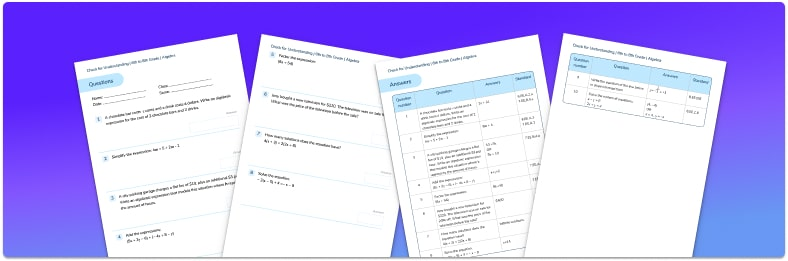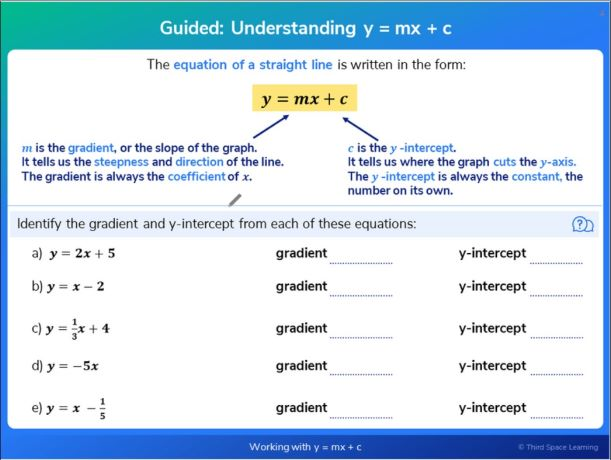Math teaching support you can trustone-on-one tutoring sessionsschools supported

[FREE] Fun Math Games & Activities Packs

Always on the lookout for fun math games and activities in the classroom? Try our ready-to-go printable packs for students to complete independently or with a partner!

# 15 Algebra Questions And Practice Problems To Do With Your Middle Schoolers

Algebra questions involve using letters or symbols to represent unknown values or values that can change. Here you will find 15 algebra questions to test your knowledge and show you the different ways that algebra can be used to solve a problem to find an unknown value or to make generalizations.Algebra Check for Understanding Quiz

Use this quiz to check your Grade 6-8 students’ understanding of algebraDownload Free Now!

### Algebra in middle school

Algebra encompasses many skills and concepts to help us make sense of the world and solve problems. In middle school, we learn to write basic algebraic expressions, write and solve linear equations, write and solve a system of linear equations, and graph linear equations on the coordinate plane. Later, we further develop these skills which allow us to work with more complex equations such as quadratic equations, polynomial equations, and exponential equations.

### How to solve algebraic equations

When you are presented with an algebraic problem, it is important to make sense of the problem. Here are some of the key terms along with what they mean:

• Solve the equation – find out the value of the unknown.
• Substitute – put the values you have been given into the algebraic expression.
• Coefficients – the amount a term has been multiplied by. For example, in the expression 4c + 2, 4 is the coefficient.
• Constants – values which are not variable and stay the same. For example, for 4c + 2, 2 is the constant.
• Binomial – a binomial or binomial expression is one which has two parts, like our example 4c + 2.
• Simplify – collect together like terms to make the expression or equation look simpler.
• Expand – multiply out the expressions inside parentheses.
• Factorize – put into parentheses.
• In terms of x – rewrite the equation in the form x = …

Remember, when working with algebra, we must still apply the order of operations, PEMDAS. i.e. Parenthesis, Exponents (powers, square roots), Multiplication, Division, Addition, Subtraction.

When working with algebraic expressions and equations we must consider carefully which operations to deal with first.

### Algebra in elementary school

Students as early as kindergarten begin to solve problems with algebraic thinking. Algebraic thinking starts with students understanding the properties of the operations, the relationships between them, and the composition of numbers.

They begin to relate concrete models to abstract expressions and equations, and continue this work throughout elementary school. They also look at patterns and the order of operations that will contribute to their understanding of algebra in later years.

This flexible understanding with the composition and representation of numbers, as well as the operations sets them up for more complex and abstract equations once they get to middle school.

### Algebra practice questions for middle school

1. A chocolate bar costs c cents and a drink costs d cents. Write down an expression for the cost of 2 chocolate bars and 2 drinks.

c+d2c+d2c+2d2c-2d2 chocolate bars would cost 2 lots of c, or 2c, and 2 drinks would cost 2 lots of d, or 2d.

2. Simplify the expression 4m+5+2m-1.

10m6m+46m+66m-4We need to collect together like terms here so 4m + 2m = 6m and 5 – 1 = 4 (watch out for the negative).

In middle school, we learn a variety of different algebra techniques to answer algebra questions and to practice problem solving with algebra. These include:

• Simplifying algebraic expressions
• Expanding brackets and factoring
• Forming algebraic equations from word problems
• Solving algebraic equations and inequalities
• Substituting into expressions
• Changing the subject of an equation
• Working with real life graphs and straight line graphs
• SequencesA slide guiding pupils through the equation of a straight line from Third Space Learning’s online intervention.

#### Algebra questions for middle school: basic algebra

1. In this pyramid, you add two adjacent blocks to find the value of the block above.What expression will be in the top box?

8a-2b12a-b12a+5b10a+b2.  Brian is a window cleaner. He uses the following formula to calculate the amount to charge his customers:

Charge = £20 + 4n

Where n is the number of windows a house has.

If a house has 7 windows, how much would Brian charge?

£24£67£48£27In this question, n is 7 so we can substitute 7 into the formula.

Charge = £20 + 4 × 7

Charge = £48

3. The area of a rectangle is 4x-6.Which of the following pairs could be the length and width of the rectangle?

4x and 6x4 and x-62 and 2x-32x and 2x-3There are two ways of attempting this question. We know that area \;of \;a \;rectangle = length × width so we could multiply each pair together to see which pair makes 4x − 6.

Alternatively, if we factorise 4x − 6 we get 2(2x − 3) meaning the sides could be 2 and 2x − 3.

4.  The formula for changing degrees Celsius to degrees Fahrenheit is:

F=\frac{9C}{5}+32

Rearrange this formula to make C the subject.

C=\frac{5(F-32)}{9}C=\frac{5F-32}{9}C=\frac{5F}{9}-32C=5F-\frac{32}{9}\begin{aligned} F&=\frac{9C}{5}+32 \hspace{3cm} &\text{subtract 32}\\\\ F-32&=\frac{9C}{5} &\text{multiply by 5}\\\\ 5(F-32)&=9C &\text{divide by 9}\\\\ \frac{5(F-32)}{9}&=C \end{aligned}

#### Algebra questions for middle school: forming and solving equations

5. Work out the size of the smallest angle.20^{\circ}26^{\circ}8^{\circ}34^{\circ}The angles in a triangle add up to 180^{\circ} therefore we can write

4x+2x-10+3x-8=180

Now we have an equation we can solve.

\begin{aligned} 9x-18&=180 \hspace{3cm} &\text{add } 18\\ 9x&=198 &\text{divide by } 9\\ x&=22^{\circ} \end{aligned}

The angles are :

\begin{aligned} 4\times22&=88^{\circ}\\ 2\times22 -10&=34^{\circ}\\ 3\times22 -8&=58^{\circ} \end{aligned}

The smallest angle is 34^{\circ} .

6.  Jamie’s dad is 4 times older than Jamie. In 14 years time, Jamie’s dad will be twice the age of Jamie.

What is the sum of Jamie’s age now and Jamie’s dad’s age now?

70422235To solve this we need to write an equation.

Let Jamie’s age now be x . Then Jamie’s dad’s age is 4x .

In 14 years time Jamie’s age will be x + 14 and Jamie’s dad’s age will be 4x + 14 .

Since we know Jamie’s dad’s age will be two times Jamie’s age, we can write

4x+14=2(x+14)

Now we have an equation we can solve.

\begin{aligned} 4x+14&=2(x+14) \hspace{3cm} &\text{expand the brackets}\\ 4x+14&=2x+28 \hspace{3cm} &\text{subtract 2x}\\ 2x+14&=28 \hspace{3cm} &\text{subtract 14}\\ 2x&=14 \hspace{3cm} &\text{expand the brackets}\\ x&=7 \hspace{3cm} \end{aligned}

Jamie is currently 7 years old meaning his dad is 28 years old. The sum of their ages is 35 .

Note: when algebraic equations contain denominators on either side, we can use the cross multiplication method to help us work out the answer. For example with the following expressions:

\frac{a}{b} = \frac{c}{d}

This can then become,

And so on.

#### Algebra questions for middle school: graphs

7.  Which of the following lines passes through the point (2, 5)?

y=2x-1y=2x+1y=4x-2y=2x+5At the point (2, 5), x is 2 and y is 5. We can check which equation works when we substitute in these values:

#### Looking for more algebra math questions?

Try these:

Please note, this blog focuses on Edexcel maths past papers, not the AQA or OCR exam boards, nor IGCSE maths. What’s more, the focus is on GCSE maths, not A Level maths or the maths Edexcel A Level.

The content in this article was originally written by secondary teacher Beki Christian and has since been revised and adapted for US schools by elementary math teacher Jaclyn Wassell.##### Beki Christian
Maths Teacher and Author
Beki has 11 years experience teaching secondary Maths. She is currently working as a Maths tutor as well as writing resources and blog posts for Third Space Learning.
x#### Algebra Check for Understanding Quiz

Use this quiz to check your Grade 6-8 students’ understanding of algebra

#### Algebra Check for Understanding QuizUse this quiz to check your Grade 6-8 students’ understanding of algebra